# M8-S8: de Broglie's and Schrödinger's Contributions to The Atomic Model

• ### investigate de Broglie’s matter waves, and the experimental evidence that developed the following formula:

- λ = h/mv (ACSPH140)

### De Broglie’s Hypothesis of Matter Waves

• Previous experiments such as the photoelectric effect and the Compton effect showed the particle-nature of light (waves).

• Louis de Broglie proposed that particles can also behave like waves in the equation:• De Broglie postulated that electrons, when orbiting the nucleus, act as standing waves (stationary waves). Standing waves are resulted from two waves travelling in opposite directions and out of phase by exactly one wavelength. This causes the two waves to superimpose and form a standing wave whose amplitude is zero. The energy of the two waves also cancel each other out.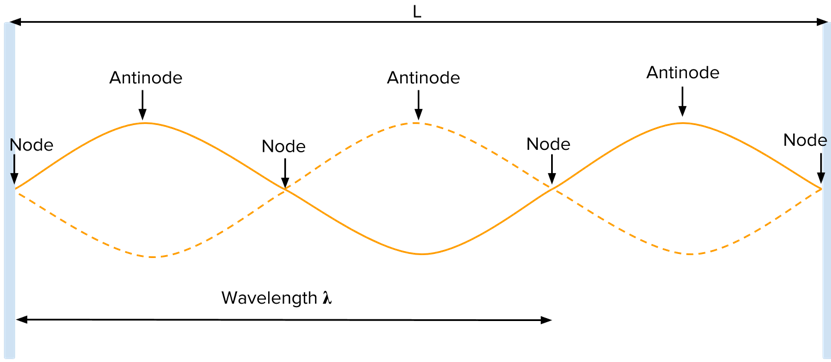A simple scenario in which a standing wave is created is when two out-of-phase waves are oscillating between two reflective surfaces.

• This hypothesis explains one of Bohr’s postulates – that electrons orbit the nucleus in stable energy states, without emitting energy. By understanding electrons’ orbits as standing waves which do not release nor absorb energy.
• This is because standing waves have a closed orbit with no movement of energy, and therefore the orbit is contained within the orbit and is therefore stable.
• De Broglie furthered his hypothesis by describing that all electron standing waves travel in quantised distances around the nucleus. In simpler words, the circumference of any orbit or energy level must be an integral multiple of the electron’s wavelength.
• Allowed energy levels or orbits are those in which an integral number of electron wavelengths exactly fit the circumference of the orbit shown below:• Thus, de Broglie’s interpretation can be expressed mathematically as 2πr=λ. The implication of this is that the number of electron wavelengths in an orbit increases with energy level. See below for a visual reference.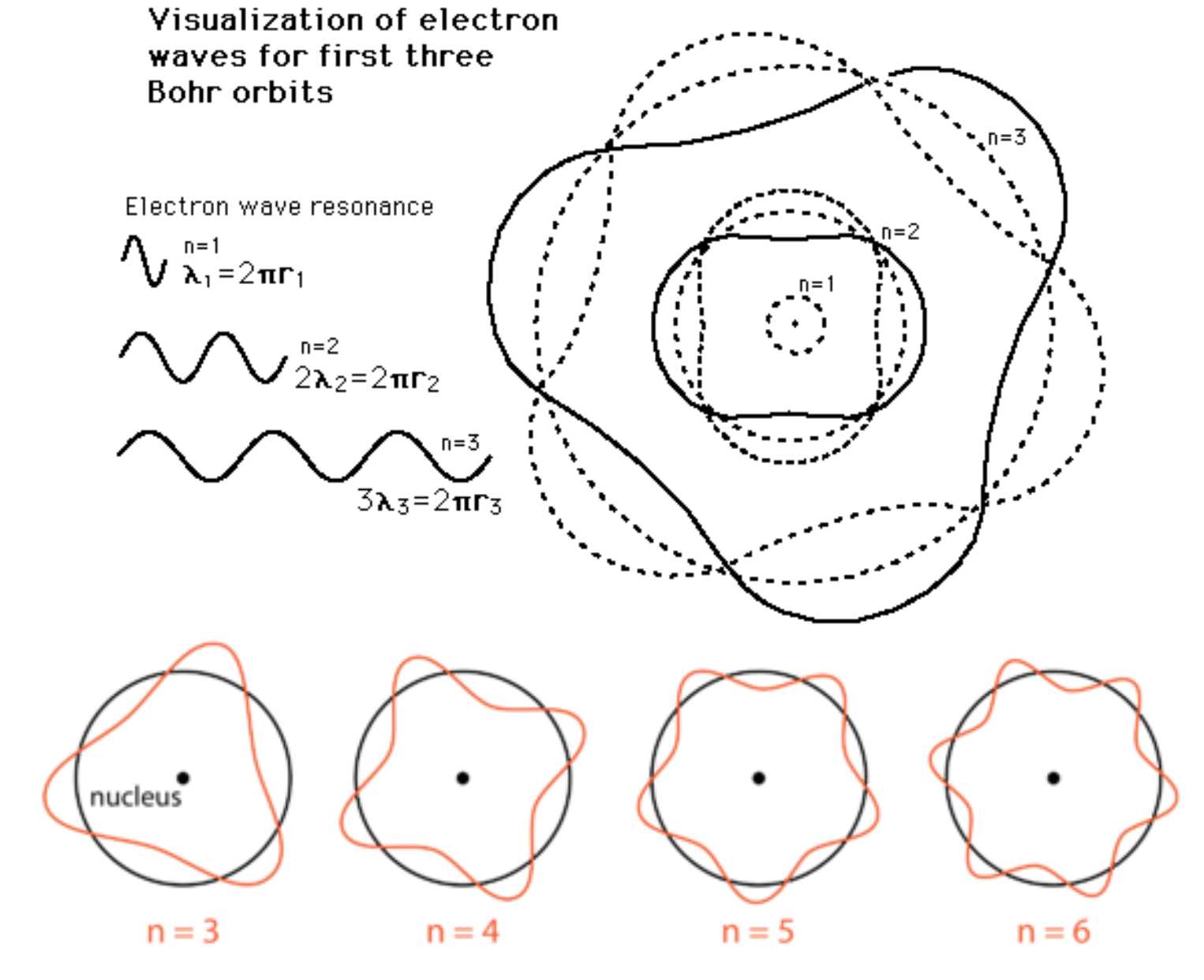﻿Figure shows changes in electron wavelength with energy level. The number of antinode (crest) corresponds to the principal quantum number (n). n simply defines the energy level.

Applying de Broglie’s matter-wave equation to this idea gives:• This expression is consistent with Bohr's third postulate - the angular momentum of an electron in a stable energy orbit is quantised.

### Particle-wave duality applies to all objects

• De Broglie’s prediction applies to particles of all sizes by their wavelengths become increasingly small as their mass become greater. For example, the de Broglie wavelength of a tennis ball is ridiculously small.
• This means the wave nature for macroscopic particles is very difficult to capture and observe.

### Experimental evidence of the wave-nature of elementary particles

• Clinton Davisson and Lester Germer were studying the surface of nickel by firing electron beams at the nickel crystal. Due to electrons' small size, they expected that this would allow them to obtain a detailed image of Nickel's surface and lattice structure.
• However, during the experiment oxygen accidentally leaked into the vacuum chamber which contained the nickel crystal. This caused the nickel to oxidise which required them to heat the nickel crystal in order to remove the oxide layer.
The details of oxidation are not required for HSC Physics
• The heating process resulted in the formation of new crystals whose lattice structural width is larger than the wavelength of an electron.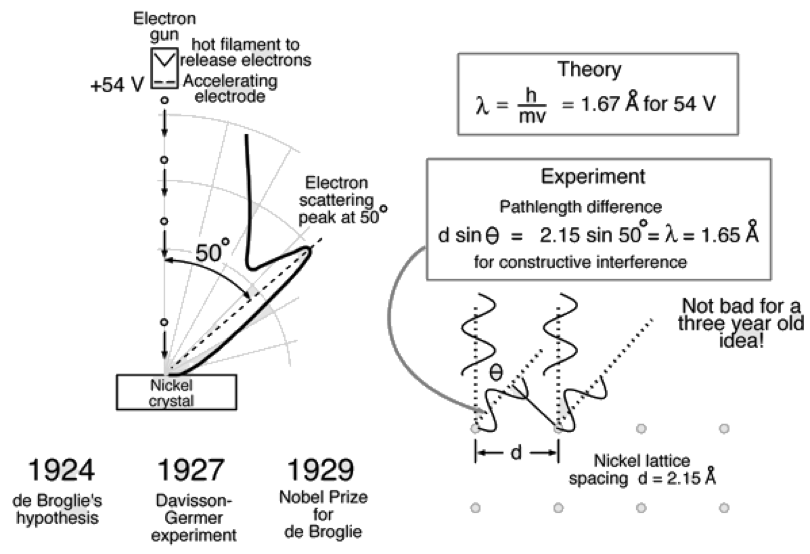• The slits between nickel atoms acted as a diffraction grating which caused electrons to diffract and therefore exhibit wave nature.
• Keep in mind that Davisson and Germer conducted this experiment two decades after J.J. Thomson's discovery of electron's charge to mass ratios. Thus, electrons were widely thought to be particles at this time.
• After observing that the first maxima of diffraction occurred at an angle of 50º to the vertical, the pair found the wavelength of diffracted electrons. The value was very close to what de Broglie had predicted.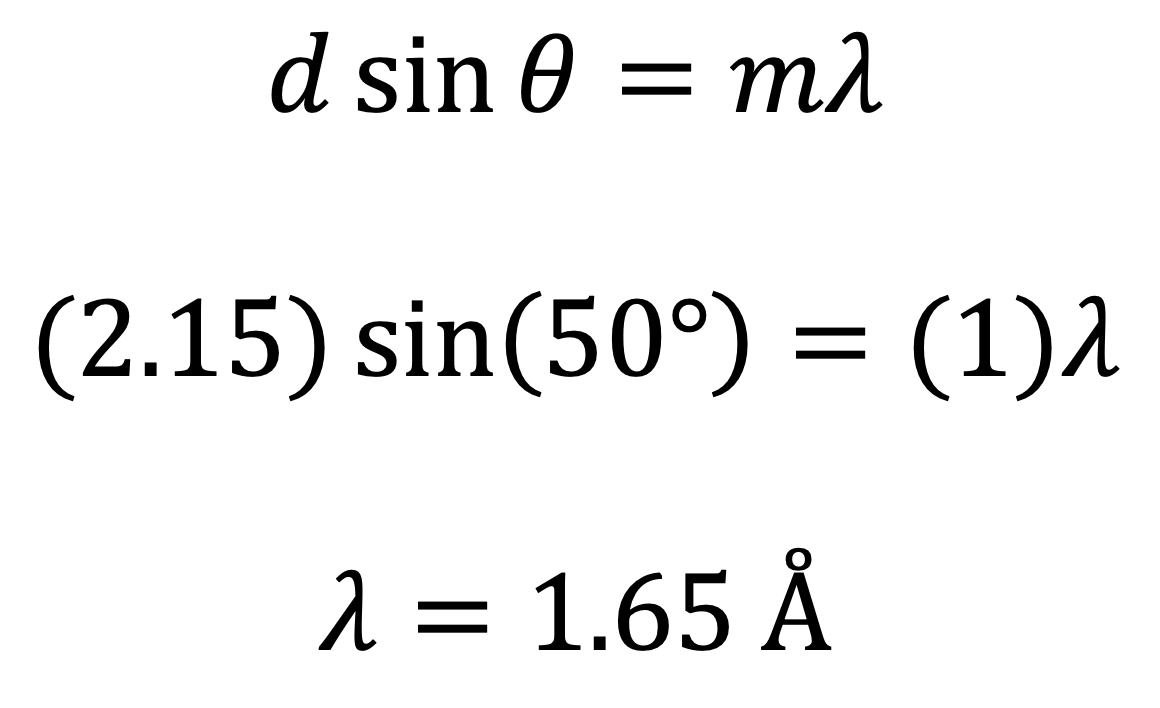• Using 2.15 Å as the width of the nickel crystal, Davisson and Germer calculated the wavelength of an electron to be 1.65 Å. This is very close the value predicted by de Broglie or 1.67 Å.

### Limitation of de Broglie's Matter Wave Theory

• Like all other theories which combine ideas from classical and quantum physics, de Broglie's wave-matter model of the atom faces the same problem.
• It is difficult to understand how electrons' physical motion allows them to adopt properties of a standing wave while being under the influence of electrostatic attraction exerted by the nucleus.

### Schrödinger’s equation and probabilistic model of the atom.

• Schrödinger used de Broglie’s electron matter wave idea to develop the probabilistic model of the atom. This is also the basis of the quantum mechanical model.
• So, instead of creating a model that focuses on defining the path and motion of electrons, Schrödinger provided a mathematical and probabilistic perspective.
• The basic form of Schrödinger’s equation is as follows: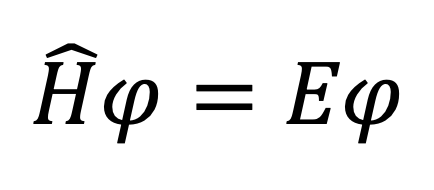where H is the Hamiltonian operator and E is the binding energy of the electron. These variables are not examined in HSC Physics. ψ is the wave function.

What you do need to know

• The solutions of the equation are wave functions with specific allowed values of E.
• The wave function squared (ψ2) reveals the density of electrons or the probability of locating an electron at a particular position relative to the nucleus. This will be discussed in more detail below.
• Unlike Bohr’s model, Schrödinger’s model interprets electrons as matter-waves and uses the property of standing waves to explain the stability of electrons’ movement in the atom.

Orbitals

• A probabilistic view of electron movement/location gives rise to orbitals. Unlike orbits, orbitals are a visual model of electron density and hence the relative probability of finding an electron in a particular region within the orbital. While 'orbits' and 'orbitals' seem to share similarities, they are very different concepts.
• Different types of orbitals have different shapes and therefore electron density patterns.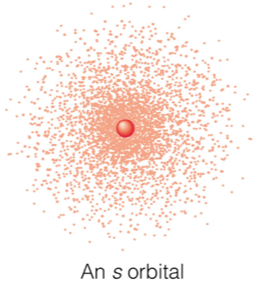Illustration of the 1s orbital depicts electron density surrounding a red nucleus in the centre. Appreciate the evident increase in probability of finding an electron with proximity to the nucleus.

• The simplest form is the s orbital which is spherical in shape.Illustrations depict different perspectives of s orbitals. Figures in the first row show a three-dimensional model of s orbitals. Appreciate the presence of nodes between s orbitals. Figures in the second row show the same features in a two-dimensional manner.

• The s orbital can also be visualised on a graph of wave function squared versus distance r away from the nucleus.
• The amplitude of each curve represents the probability. As seen, the probability decreases with subsequent s orbitals as they become further away from the nucleus.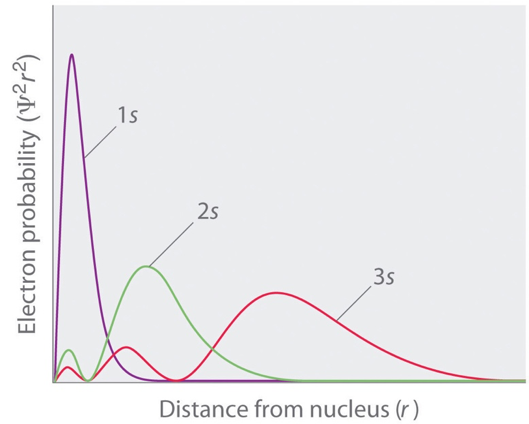Wave function squared versus distance from the nucleus for s orbitals.• The angular momentum of electrons gives rise to other types of orbitals (p, d and f) all of which are not examined in detail in HSC Physics.

Practice Question 1

Calculate the de Broglie wavelength of an electron travelling at 0.2 c in a particle accelerator.

Practice Question 2

Discuss the contribution from de Broglie in the development of a valid atomic model (4 marks)

Practice Question 3

Compare Schrodinger's atomic model to that of Niels Bohr's. (4 marks)

Previous section: Rydberg's Equation

﻿Next section: ﻿Introduction to Radioactive Decay (Theory)